Anzeige

# HERMITE SERIES

25. Dec 2020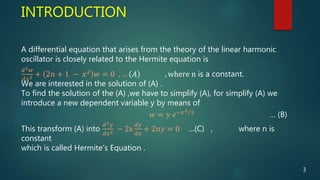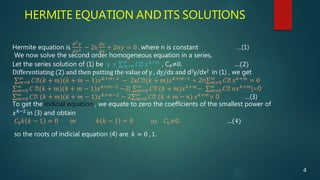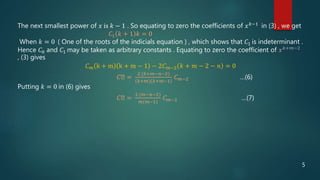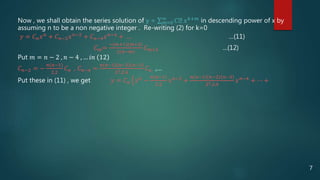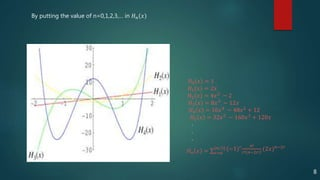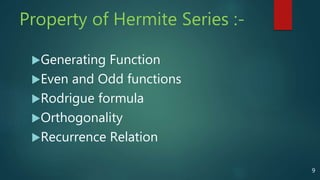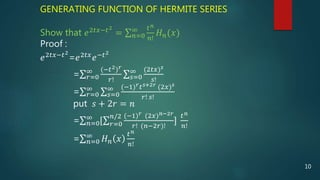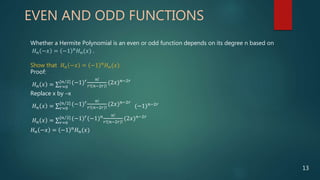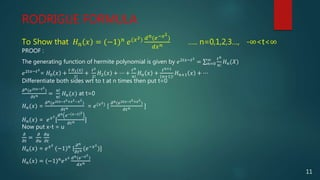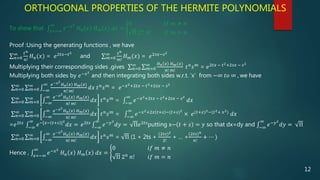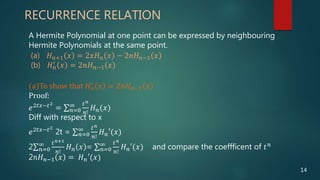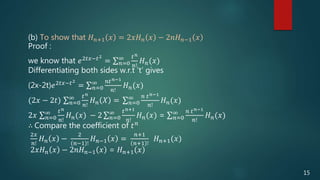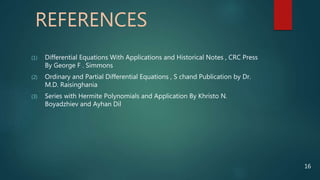1 von 16
Anzeige

### HERMITE SERIES

1. CONTENTS  INTRODUCTION  HERMITE EQUATION AND ITS SOLUTIONS  PROPERTY OF HERMITE SERIES * GENERATING FUNCTION * RODRIGUE FORMULA * ORTHOGONALITY * EVEN AND ODD FUNCTIONS * RECURRENCE RELATION  REFERENCES 2
2. A differential equation that arises from the theory of the linear harmonic oscillator is closely related to the Hermite equation is 𝑑2 𝑤 𝑑𝑥2 + 2𝑛 + 1 − 𝑥2 𝑤 = 0 , … 𝐴 , where n is a constant. We are interested in the solution of (A) . To find the solution of the (A) ,we have to simplify (A), for simplify (A) we introduce a new dependent variable y by means of 𝑤 = 𝑦 𝑒−𝑥2 2 … (B) This transform (A) into 𝑑2 𝑦 𝑑𝑥2 − 2x 𝑑𝑦 𝑑𝑥 + 2𝑛𝑦 = 0 …(C) , where n is constant which is called Hermite’s Equation . INTRODUCTION 3
3. HERMITE EQUATION AND ITS SOLUTIONS Hermite equation is 𝑑2 𝑦 𝑑𝑥2 − 2x 𝑑𝑦 𝑑𝑥 + 2𝑛𝑦 = 0 , where n is constant …(1) We now solve the second order homogeneous equation in a series, Let the series solution of (1) be y = 𝑚=0 ∞ 𝐶ₘ 𝑥 𝑘+𝑚 , C₀≠0. …(2) Differentiating (2) and then putting the value of y , dy/dx and d2y/dx2 in (1) , we get 𝑚=0 ∞ 𝐶ₘ(𝑘 + 𝑚)(𝑘 + 𝑚 − 1)𝑥 𝑘+𝑚−2 − 2x𝐶ₘ(𝑘 + 𝑚)𝑥 𝑘+𝑚−1 + 2n 𝑚=0 ∞ 𝐶ₘ 𝑥 𝑘+𝑚 = 0 𝑚=0 ∞ 𝐶 ₘ(𝑘 + 𝑚)(𝑘 + 𝑚 − 1)𝑥 𝑘+𝑚−2 −2[ 𝑚=0 ∞ 𝐶ₘ (𝑘 + 𝑚)𝑥 𝑘+𝑚− 𝑚=0 ∞ 𝐶ₘ 𝑛𝑥 𝑘+𝑚]=0 𝑚=0 ∞ 𝐶ₘ (𝑘 + 𝑚)(𝑘 + 𝑚 − 1)𝑥 𝑘+𝑚−2 − 2 𝑚=0 ∞ 𝐶ₘ (𝑘 + 𝑚 − 𝑛) 𝑥 𝑘+𝑚 = 0 …(3) To get the indicial equation , we equate to zero the coefficients of the smallest power of 𝑥 𝑘−2 in (3) and obtain 𝐶0 𝑘 𝑘 − 1 = 0 or 𝑘 𝑘 − 1 = 0 𝑎𝑠 𝐶0≠0. …(4) so the roots of indicial equation (4) are 𝑘 = 0 , 1. 4
4. The next smallest power of 𝑥 is 𝑘 − 1 . So equating to zero the coefficients of 𝑥 𝑘−1 in (3) , we get 𝐶1 𝑘 + 1 𝑘 = 0 When 𝑘 = 0 ( One of the roots of the indicials equation ) , which shows that 𝐶1 is indeterminant . Hence 𝐶0 and 𝐶1 may be taken as arbitrary constants . Equating to zero the coefficient of 𝑥 𝑘+𝑚−2 , (3) gives 𝐶 𝑚 k + m k + m − 1 − 2𝐶 𝑚−2 𝑘 + 𝑚 − 2 − 𝑛 = 0 𝐶ₘ = 2 (𝑘+𝑚−𝑛−2) (𝑘+𝑚)(𝑘+𝑚−1) 𝐶 𝑚−2 …(6) Putting 𝑘 = 0 in (6) gives 𝐶ₘ = 2 (𝑚−𝑛−2) 𝑚(𝑚−1) 𝐶 𝑚−2 …(7) 5
5. put m=2,4,6,…,2m in (7), we have 𝐶2= −2𝑛 2! 𝐶0 𝐶4= −1 222 𝑛(𝑛−2) 4! 𝐶0 … 𝐶2𝑚 = −1 𝑚2 𝑚 𝑛 𝑛−2 …(𝑛−2𝑚+2) 2𝑚! Put m=3,5,7,…,2m+1 in (7) , we have 𝐶3= −1 121(𝑛−1) 3! 𝐶1 𝐶5= −1 222(𝑛−1)(𝑛−3) 5! 𝐶1 … 𝐶2𝑚+1= −1 𝑚2 𝑚 𝑛−1 𝑛−3 …(𝑛−2𝑚+1) 2𝑚+1 ! 𝐶1 Now put above values in (2) with k=0 , we get 𝑦 = 𝐶0 + 𝐶1 𝑥 + 𝐶2 𝑥2 + 𝐶3 𝑥3 + … 𝑦 = 𝐶0[1 − 2𝑛 2! 𝑥2 + −1 222 𝑛(𝑛−2) 4! 𝑥4 + ⋯ + −1 𝑚2 𝑚 𝑛 𝑛−2 … 𝑛−2𝑚+2 2𝑚! 𝑥2𝑚] + 𝐶1[𝑥 − 21 𝑛−1 3! 𝑥3 + 22 𝑛−1 𝑛−3 5! 𝑥5 + ⋯ + −1 𝑚2 𝑚 𝑛−1 𝑛−3 … 𝑛−2𝑚+1 2𝑚+1 ! 𝑥2𝑚+1] 𝑦 = 𝐶0 𝑢 + 𝐶1v Replacing m by m+2 in (7) , 𝐶 𝑚+2 = 2(𝑚−𝑛) (𝑚+1)(𝑚+2) 𝐶 𝑚 …(10) 6
6. Now , we shall obtain the series solution of y = 𝑚=0 ∞ 𝐶ₘ 𝑥 𝑘+𝑚 in descending power of x by assuming n to be a non negative integer . Re-writing (2) for k=0 𝑦 = 𝐶 𝑛 𝑥 𝑛 + 𝐶 𝑛−2 𝑥 𝑛−2 + 𝐶 𝑛−4 𝑥 𝑛−4 + … …(11) 𝐶 𝑚= −(𝑚+1)(𝑚+2) 2(𝑛−𝑚) 𝐶 𝑚+2 …(12) Put 𝑚 = 𝑛 − 2 , 𝑛 − 4 , … 𝑖𝑛 12 𝐶 𝑛−2 = − 𝑛(𝑛−1) 2.2 𝐶 𝑛 , 𝐶 𝑛−4 = 𝑛(𝑛−1)(𝑛−2)(𝑛−3) 22.2.4 𝐶 𝑛 ,… Put these in (11) , we get 𝑦 = 𝐶 𝑛 𝑥 𝑛 − 𝑛 𝑛−1 2.2 𝑥 𝑛−2 + 𝑛 𝑛−1 𝑛−2 𝑛−3 22.2.4 𝑥 𝑛−4 + ⋯ + 7
7. 𝐻0 𝑥 = 1 𝐻1 𝑥 = 2𝑥 𝐻2 𝑥 = 4𝑥2 − 2 𝐻3 𝑥 = 8𝑥3 − 12𝑥 𝐻4 𝑥 = 16𝑥4 − 48𝑥2 + 12 𝐻5 𝑥 = 32𝑥5 − 160𝑥3 + 120𝑥 . . . 𝐻 𝑛 𝑥 = 𝑟=0 [ 𝑛 2] (−1) 𝑟 𝑛! 𝑟! 𝑛−2𝑟 ! (2𝑥) 𝑛−2𝑟 By putting the value of n=0,1,2,3,… in 𝐻 𝑛 𝑥 8
8. Property of Hermite Series :- Generating Function Even and Odd functions Rodrigue formula Orthogonality Recurrence Relation 9
9. GENERATING FUNCTION OF HERMITE SERIES Show that 𝑒2𝑡𝑥−𝑡2 = 𝑛=0 ∞ 𝑡 𝑛 𝑛! 𝐻 𝑛(𝑥) Proof : 𝑒2𝑡𝑥−𝑡2 =𝑒2𝑡𝑥 𝑒−𝑡2 = 𝑟=0 ∞ (−𝑡2) 𝑟 𝑟! 𝑠=0 ∞ (2𝑡𝑥) 𝑠 𝑠! = 𝑟=0 ∞ 𝑠=0 ∞ (−1) 𝑟 𝑡 𝑠+2𝑟 (2𝑥) 𝑠 𝑟! 𝑠! put 𝑠 + 2𝑟 = 𝑛 = 𝑛=0 ∞ [ 𝑟=0 𝑛/2 (−1) 𝑟 (2𝑥) 𝑛−2𝑟 𝑟! (𝑛−2𝑟)! ] 𝑡 𝑛 𝑛! = 𝑛=0 ∞ 𝐻 𝑛 𝑥 𝑡 𝑛 𝑛! 10
10. EVEN AND ODD FUNCTIONS Whether a Hermite Polynomial is an even or odd function depends on its degree n based on 𝐻 𝑛 −𝑥 = −1 𝑛 𝐻 𝑛(𝑥) . Show that 𝐻 𝑛 −𝑥 = −1 𝑛 𝐻 𝑛(𝑥) Proof: 𝐻 𝑛 𝑥 = 𝑟=0 [ 𝑛 2] (−1) 𝑟 𝑛! 𝑟! 𝑛−2𝑟 ! (2𝑥) 𝑛−2𝑟 Replace x by –x 𝐻 𝑛 𝑥 = 𝑟=0 [ 𝑛 2] (−1) 𝑟 𝑛! 𝑟! 𝑛−2𝑟 ! (2𝑥) 𝑛−2𝑟 (−1) 𝑛−2𝑟 𝐻 𝑛 𝑥 = 𝑟=0 [ 𝑛 2] (−1) 𝑟 (−1) 𝑛 𝑛! 𝑟! 𝑛−2𝑟 ! (2𝑥) 𝑛−2𝑟 𝐻 𝑛 −𝑥 = −1 𝑛 𝐻 𝑛(𝑥) 13
11. RODRIGUE FORMULA To Show that 𝐻 𝑛 𝑥 = (−1) 𝑛 𝑒(𝑥2) 𝑑 𝑛(𝑒−𝑥2 ) 𝑑𝑥 𝑛 ….. n=0,1,2,3…, -∞<t<∞ PROOF : The generating function of hermite polynomial is given by 𝑒2𝑡𝑥−𝑡2 = 𝑛=0 ∞ 𝑡 𝑛 𝑛! 𝐻 𝑛(𝑋) 𝑒2𝑡𝑥−𝑡2 = 𝐻0 𝑥 + 𝑡 𝐻1(𝑥) 1! + 𝑡2 2! 𝐻2 𝑥 + ⋯ + 𝑡 𝑛 𝑛! 𝐻 𝑛 𝑥 + 𝑡 𝑛+1 𝑛+1 ! 𝐻 𝑛+1 𝑥 + ⋯ Differentiate both sides wrt to t at n times then put t=0 𝜕 𝑛(𝑒2𝑡𝑥−𝑡2 ) 𝜕𝑡 𝑛 = 𝑛! 𝑛! 𝐻 𝑛(𝑥) at t=0 𝐻 𝑛(𝑥) = 𝜕 𝑛(𝑒2𝑡𝑥−𝑡2+𝑥2−𝑥2 ) 𝜕𝑡 𝑛 = 𝑒(𝑥2) [ 𝜕 𝑛(𝑒2𝑡𝑥−𝑡2+𝑥2 ) 𝜕𝑡 𝑛 ] 𝐻 𝑛(𝑥) = 𝑒 𝑥2 [ 𝜕 𝑛 𝑒− 𝑥−𝑡 2 𝜕𝑡 𝑛 ] Now put x-t = u 𝜕 𝜕𝑡 = 𝜕 𝜕𝑢 𝜕𝑢 𝜕𝑡 𝐻 𝑛(𝑥) = 𝑒 𝑥2 (−1) 𝑛 [ 𝜕 𝑛 𝜕𝑡 𝑛 (𝑒−𝑥2 )] 𝐻 𝑛 𝑥 = (−1) 𝑛 𝑒 𝑥2 𝑑 𝑛(𝑒−𝑥2 ) 𝑑𝑥 𝑛 11
12. ORTHOGONAL PROPERTIES OF THE HERMITE POLYNOMIALS To show that 𝑥=−∞ ∞ 𝑒−𝑥2 𝐻 𝑛 𝑥 𝐻 𝑚 𝑥 𝑑𝑥 = 0 𝑖𝑓 𝑚 ≠ 𝑛 П 2 𝑛 𝑛! 𝑖𝑓 𝑚 = 𝑛 Proof :Using the generating functions , we have 𝑛=0 ∞ 𝑡 𝑛 𝑛! 𝐻 𝑛(𝑥) = 𝑒2𝑡𝑥−𝑡2 and 𝑚=0 ∞ 𝑠 𝑛 𝑚! 𝐻 𝑚(𝑥) = 𝑒2𝑠𝑥−𝑠2 Multiplying their corresponding sides ,gives 𝑛=0 ∞ 𝑚=0 ∞ 𝐻 𝑛 𝑥 𝐻 𝑚(𝑥) 𝑛! 𝑚! 𝑡 𝑛 𝑠 𝑚 = 𝑒2𝑡𝑥 − 𝑡2+2𝑠𝑥 − 𝑠2 Multiplying both sides by 𝑒−𝑥2 and then integrating both sides w.r.t. ‘x’ from −∞ 𝑡𝑜 ∞ , we have 𝑛=0 ∞ 𝑚=0 ∞ −∞ ∞ 𝑒−𝑥2 𝐻 𝑛 𝑥 𝐻 𝑚(𝑥) 𝑛! 𝑚! 𝑑𝑥 𝑡 𝑛 𝑠 𝑚 = 𝑒−𝑥2+2𝑡𝑥 − 𝑡2+2𝑠𝑥 − 𝑠2 𝑛=0 ∞ 𝑚=0 ∞ −∞ ∞ 𝑒−𝑥2 𝐻 𝑛 𝑥 𝐻 𝑚(𝑥) 𝑛! 𝑚! 𝑑𝑥 𝑡 𝑛 𝑠 𝑚 = −∞ ∞ 𝑒−𝑥2+2𝑡𝑥 − 𝑡2+2𝑠𝑥 − 𝑠2 𝑑𝑥 𝑛=0 ∞ 𝑚=0 ∞ −∞ ∞ 𝑒−𝑥2 𝐻 𝑛 𝑥 𝐻 𝑚(𝑥) 𝑛! 𝑚! 𝑑𝑥 𝑡 𝑛 𝑠 𝑚 = −∞ ∞ 𝑒−𝑥2+2𝑥 𝑡+𝑠 −(𝑡+𝑠)2 × 𝑒(𝑡+𝑥)2−(𝑡2+ 𝑥2) 𝑑𝑥 =𝑒2𝑡𝑠 −∞ ∞ 𝑒−[𝑥− 𝑡+𝑠 ]2 𝑑𝑥 = 𝑒2𝑡𝑠 −∞ ∞ 𝑒−𝑦2 𝑑𝑦 = П𝑒2𝑡𝑠 putting x− 𝑡 + 𝑠 = 𝑦 so that dx=dy and −∞ ∞ 𝑒−𝑦2 𝑑𝑦 = П 𝑛=0 ∞ 𝑚=0 ∞ −∞ ∞ 𝑒−𝑥2 𝐻 𝑛 𝑥 𝐻 𝑚(𝑥) 𝑛! 𝑚! 𝑑𝑥 𝑡 𝑛 𝑠 𝑚 = П (1 + 2ts + (2𝑡𝑠)2 2! + … + (2𝑡𝑠) 𝑛 𝑛! + ⋯ ) Hence , 𝑥=−∞ ∞ 𝑒−𝑥2 𝐻 𝑛 𝑥 𝐻 𝑚 𝑥 𝑑𝑥 = 0 𝑖𝑓 𝑚 ≠ 𝑛 П 2 𝑛 𝑛! 𝑖𝑓 𝑚 = 𝑛 12
13. RECURRENCE RELATION A Hermite Polynomial at one point can be expressed by neighbouring Hermite Polynomials at the same point. (a) 𝐻 𝑛+1 𝑥 = 2𝑥𝐻 𝑛 𝑥 − 2𝑛𝐻 𝑛−1 𝑥 (b) 𝐻 𝑛 ′ 𝑥 = 2𝑛𝐻 𝑛−1 𝑥 (a)To show that 𝐻 𝑛 ′ 𝑥 = 2𝑛𝐻 𝑛−1 𝑥 Proof: 𝑒2𝑡𝑥−𝑡2 = 𝑛=0 ∞ 𝑡 𝑛 𝑛! 𝐻 𝑛(𝑥) Diff with respect to x 𝑒2𝑡𝑥−𝑡2 2t = 𝑛=0 ∞ 𝑡 𝑛 𝑛! 𝐻 𝑛′(𝑥) 2 𝑛=0 ∞ 𝑡 𝑛+1 𝑛! 𝐻 𝑛 𝑥 = 𝑛=0 ∞ 𝑡 𝑛 𝑛! 𝐻 𝑛′(𝑥) and compare the coeffficent of 𝑡 𝑛 2𝑛𝐻 𝑛−1 𝑥 = 𝐻 𝑛′(𝑥) 14
14. (b) To show that 𝐻 𝑛+1 𝑥 = 2𝑥𝐻 𝑛 𝑥 − 2𝑛𝐻 𝑛−1 𝑥 Proof : we know that 𝑒2𝑡𝑥−𝑡2 = 𝑛=0 ∞ 𝑡 𝑛 𝑛! 𝐻 𝑛(𝑥) Differentiating both sides w.r.t ‘t’ gives (2x-2t)𝑒2𝑡𝑥−𝑡2 = 𝑛=0 ∞ 𝑛𝑡 𝑛−1 𝑛! 𝐻 𝑛(𝑥) (2𝑥 − 2𝑡) 𝑛=0 ∞ 𝑡 𝑛 𝑛! 𝐻 𝑛 𝑋 = 𝑛=0 ∞ 𝑛 𝑡 𝑛−1 𝑛! 𝐻 𝑛(𝑥) 2𝑥 𝑛=0 ∞ 𝑡 𝑛 𝑛! 𝐻 𝑛(𝑥) − 2 𝑛=0 ∞ 𝑡 𝑛+1 𝑛! 𝐻 𝑛(𝑥) = 𝑛=0 ∞ 𝑛 𝑡 𝑛−1 𝑛! 𝐻 𝑛(𝑥) ∴ Compare the coefficient of 𝑡 𝑛 2𝑥 𝑛! 𝐻 𝑛 𝑥 − 2 𝑛−1 ! 𝐻 𝑛−1 𝑥 = 𝑛+1 𝑛+1 ! 𝐻 𝑛+1(𝑥) 2𝑥𝐻 𝑛 𝑥 − 2𝑛𝐻 𝑛−1 𝑥 = 𝐻 𝑛+1 𝑥 15
15. REFERENCES (1) Differential Equations With Applications and Historical Notes , CRC Press By George F . Simmons (2) Ordinary and Partial Differential Equations , S chand Publication by Dr. M.D. Raisinghania (3) Series with Hermite Polynomials and Application By Khristo N. Boyadzhiev and Ayhan Dil 16
16. THANK YOU … 17
Anzeige